Author: Romain Vergne (website)
Please cite my name and add a link to my web page if you use this course

# Image synthesis and OpenGL: exercices 04

## Setup

We will use Linux and the following libraries:

Installing sources. In a terminal, do:
2. If needed, edit the file main.pro to change paths
3. To compile: qmake && make
4. To run: ./tp04 models/file.off
5. To edit: use either your prefered text editor or qtcreator

## Local Lighting

The goal of these exercises is to familiarize yourself with local lighting effects. You should obtain something like this: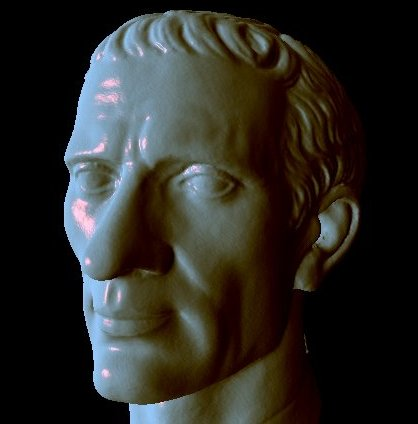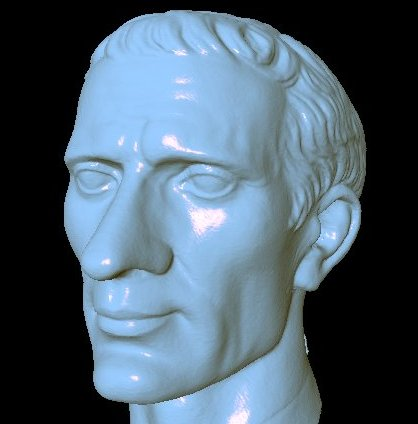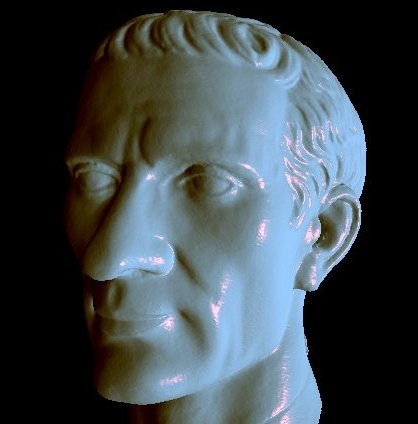### Exercice 1: Understand the code

The source code contains the same files/classes as before
• camera
By default, the program loads a mesh and uses a simple shader to display it.
Output colors are normals in camera space.
The mesh is sent to the graphics card using vertex arrays objects, like in the previous assignment.

Have a look at the files viewer.* and shaders/constant.*
You should now have an idea of the effect of each function in theses files.

### Exercice 2: Prepare a new shader

1. Create new shader files called gouraud1.vert et gouraud1.frag
1. you can simply copy/rename/paste the constant.vert and frag files
• Note: Pressing the space bar has the effect of activating the next shader in the shader list
• Note: the variable _light contains a light direction updated when the right mouse button is pressed
• Note: you will need to use glGetAttribLocation and glUniform, as for matrices (except that you send a vector3D here)

### Exercice 3: Gouraud Shading and lambertian model

Observe that 3 matrices are sent to the shaders:
• The modelview matrix
• The projection matrix
• The normal matrix
• This matrix allows to transform the normal from world space to view space
• Why not using the top left 3x3 submatrix of the modelview one?
• normalView = vec3(mdv*vec4(normalWorld,0));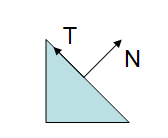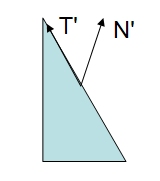Uniform scaling: no problem Non-uniform scaling: The obtained normal does not correspond anymore to the real surface
• Solution: normal matrix = transpose(inverse(upperleft3x3(modelview matrix)))
• It avoids non-uniform scaling effects that can appear in the modelview matrix
1. Apply a Gouraud shading (lighting per vertex), using the lambertian model
• Note: the lambertian model is given by: $$\rho_d \mathbf{n}\cdot\pmb{\ell})$$ where $$\rho_d$$ is a surface color (your choice in the shader)
• Note: All these operations may be done in view space

### Exercice 4: Gouraud Shading and Phong model

2. Modify the shaders to apply a Gouraud shading using the Phong model
• Note: the Phong model is given by:  $$\rho_a + \rho_{d} (\mathbf{n}\cdot\pmb{\ell}) + \rho_{s} (\mathbf{r}\cdot\mathbf{e})^{ec}$$, where
• $$\rho_a$$ is a surface ambient color (your choice)
• $$\rho_d$$ is a surface diffuse color (your choice)
• $$\rho_s$$ is a surface specular color (your choice)
• $$ec$$ is an exponent coeficient that controls the size and sharpness of the highlights
• Note: you can use the key 'r' to test and reload shaders when a change is made